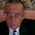### Optimization: Simplex Algorithm

Imagine we have a set of computers running simulations. Those computers can be described by a group of features, for example its power consumption per hour and the total number of processes it can run per hour. From our R+D personnel, a series of programs are send to these computers so we have to perform some schedule to run the applications, but for our profit we wish to minimise the total consumption of the set of machines. Let's show some more detail:

• Computer 1 has a power consumption of 100 watts per hour and can run 10  high cpu-demanding processes per hour and 7 low cpu-demanding processes per hour at the same time.
• Computer 2 has a power consumption of 350 watts per hour and can run 60 high cpu-demanding processes per hour and 20 low cpu-demanding processes per hour at the same time.
• From the R+D area there are a set of at least 3125 high cpu-demanding processes and at least 710 low cpu-demanding processes to be processed.

How many hours should our set of computers run to minimise the power consumption but meeting the restrictions of our R+D personnel?

This is a typical example of an optimisation problem, that is, a problem consisting in the selection of a best element with regard to some criteria from some set of variable alternatives. Firstly, we have to rewrite our problem in a more convenient way. We have to introduce two variables, the time (in hours) of the first computer and the time (also in hours) of the second computer, represented respectively by the variables x and y:

minimise  F = 100 x + 350 y  (we call this the objective function)

subject to 10 x + 60 y >= 3125, (we call these the restrictions)
7 x + 20 y  >= 710,
x >= 0, y >= 0.

So, from our Computer Science point of view, what can we do to solve this kind problems? The answer is 'many things' so the purpose of this post is to introduce a clever algorithm called Simplex.

Simplex solves Linear Programming problems (i.e., those in which the objective function to minimise---or maximise---is linear) and does it by generating a tableau and cleverly operating with it until some exit criteria is met. This algorithm is summarised as follows: the restriction equations are set in the tableau in the first place and then the objective function in the last row of this tableau. Next, because we want a minimisation, we transpose this tableau and we augment it by adding slack variables (i.e., new variables that allow us to get rid of the inequalities), one per restriction.  After this, a series of operations are performed in order to obtain the desired solution. In what follows, the main loop of the algorithm is briefly described:

(1) Check for the pivot column by looking for the greatest negative value in all the rows of the tableau.

(2) Select the pivot row by dividing the elements of the pivot column by the last column of the tableau (the different   values of the equation). The selected row will be the one containing the column value which has the lowest non-negative.

(3) Divide the entire pivot row by the value selected in the second step.

(4) Form zeroes in the values of the non-slack variables by combining multiples of the pivot row and the rest of rows (not including the pivot one).

(5) If the values of all the cells of the objective function row in the tableau are less than zero, jump to step 1. Otherwise stop.

Finally, the values of the minimisation are collected. The minimum value is in the last column of the last row of the tableau. The values of the variables (i.e., x and y in the example) for this minimum are in the objective function row cells, more precisely in the slack variables.

The optimal solution is a minimum power consumption of (F) 18229.167 watts, with a usage of the first computer of (x) 0 hours and a usage of the second computer of (y) 52.083 hours.

That's a very pretty clever and straightforward algorithm to solve linear problems. But we can go beyond with other techniques, for example using metaheuristics, but this will be a future post.

1.Remember the familiar ( c_{j} − z_{j} ) coefficients of the zero-row of the Simplex Tableau. Let k(i) be the function returning the index of the Departing Variable when the Pivot in on the i.th row. Then

z_{j} = ∑ c_{k(i)} y_{i, j} where the summation is over i=1, ..., m; and y_{i, j}'s are Tableau entries.

Can anybody prove this? I cannot find a numerical counter-example.